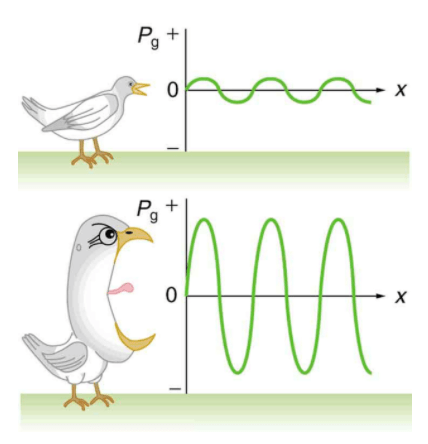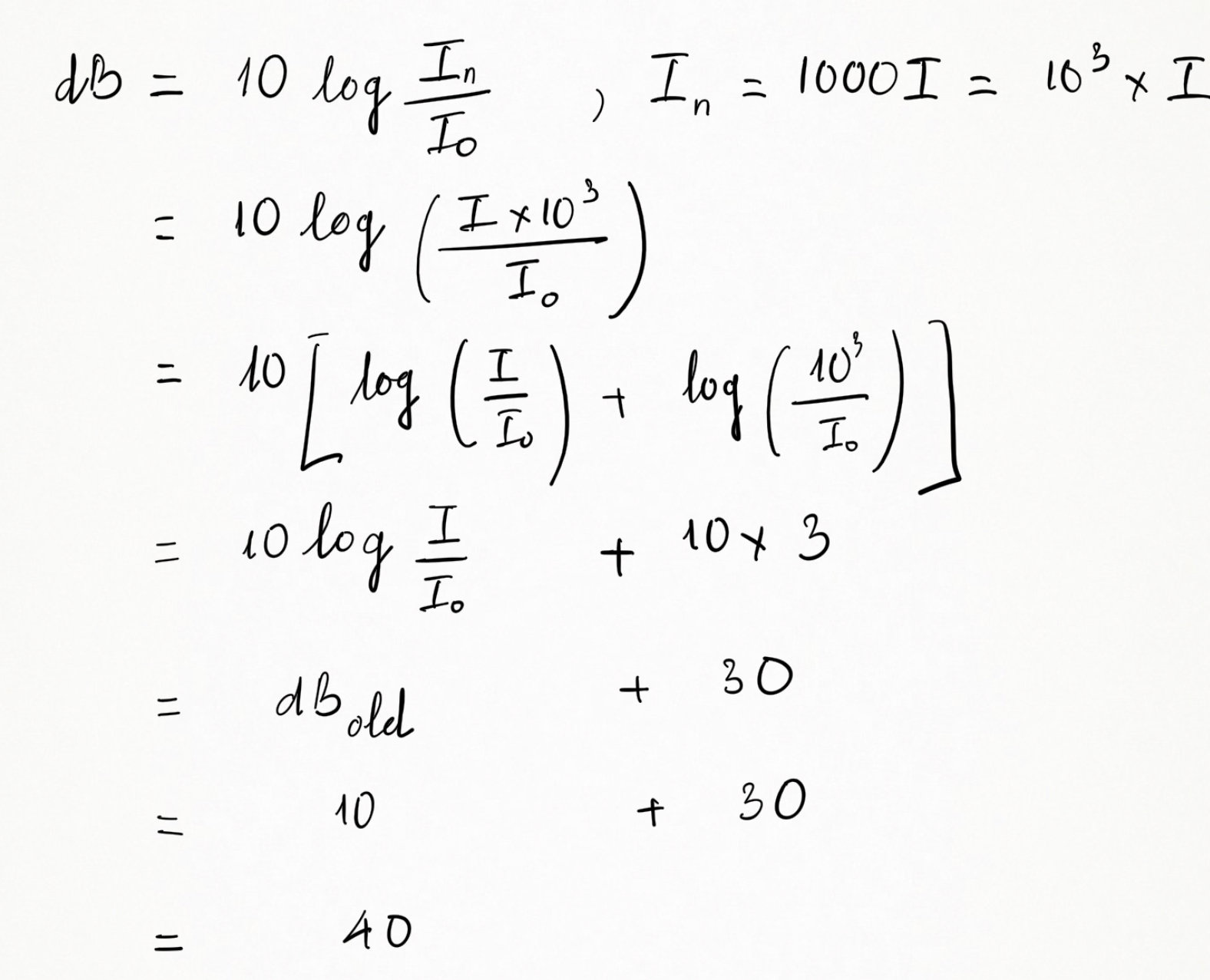Having trouble writing your personal statement?

MCAT Content / Sound / Intensity Of Sound Decibel Units Log Scale

### Intensity of Sound, Decibel units, Log Scale

Topic: Sound

Sound Intensity is the power per unit area carried by a wave; power is the rate that energy is transferred by a wave.

Sound Intensity is the power per unit area carried by a wave. Power is the rate that energy is transferred by a wave. The equation used to calculate this intensity, I, is: I = P/A. P is the power going through the area, A. The SI unit for intensity is watts per meter squared or W/m2. This is the general intensity formula, but let’s look at it from a sound perspective.

Sound intensity can be found from the following equation:– Δp – change in pressure, or amplitude
– ρ – density of the material the sound is traveling through
– vw – speed of observed soundNow we have a way to calculate the sound intensity, so let’s talk about observed intensity. The pressure variation, amplitude, is proportional to the intensity, So it is safe to say that the larger your sound wave oscillation, the more intense your sound will be. This figure shows this concept.The more energy the sound wave has, it has more energy and the louder it is to human’s ear. Although the units for sound intensity are technically watts per meter squared, it is much more common for it to be referred to as decibels, dB. A decibel is a ratio of the observed amplitude, or intensity level to a reference, which is 0 dB. The equation for this is:A more practical way to deal with intensity is to utilize the log scale. We can observe this through an example: Imagine we have a sound that is a 10 Db. What is the Decibel reading if we make it 1000 times louder.

In this example, we are not changing the Base amount (Io), but are making changes to the actual intensity.Every ten times (x10) increase in intensity translates to plus ten (+10) in the Decibel scale. So (+20) on the Decibel scale means the sound intensity increases (10×10 = 100 times).

Practice Questions

How movements influence an ultrasound

MCAT Official Prep (AAMC)

Physics Question Pack Question 42

Physics Question Pack Question 59

Key Points

• Sound intensity can be found from the following equation:Δ p – change in pressure, or amplitude
ρ – density of the material the sound is traveling through
vw – speed of observed sound.

• The larger your sound wave oscillation, the more intense your sound will be.

• Although the units for sound intensity are technically watts per meter squared, it is much more common for it to be referred to as decibels, dB.

Key Terms

decibel: A common measure of sound intensity that is one-tenth of a bel on the logarithmic intensity scale. It is defined aswhere P1 and P2 are the relative powers of the sound.

amplitude: The maximum absolute value of some quantity that varies.

Billing Information